International
Tables for
Crystallography
Volume C
Mathematical, physical and chemical tables
Edited by E. Prince

International Tables for Crystallography (2006). Vol. C, ch. 4.4, pp. 443-444

## Section 4.4.3. Resolution functions

R. Pynnd and J. M. Rowee

### 4.4.3. Resolution functions

| top | pdf |

In a Gedanken neutron scattering experiment, neutrons of wavevector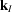impinge on a sample and the wavevector,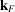, of the scattered neutrons is determined. A number of different types of spectrometer are used to achieve this goal (cf. Pynn, 1984). In each case, finite instrumental resolution is a result of uncertainties in the definition ofand. Propagation directions for neutrons are generally defined by Soller collimators for which the transmission as a function of divergence angle generally has a triangular shape. Neutron monochromatization may be achieved either by Bragg reflection from a (usually) mosaic crystal or by a time-of-flight method. In the former case, the mosaic leads to a spread of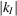while, in the latter, pulse length and uncertainty in the lengths of flight paths (including sample size and detector thickness) produce a similar effect. Calculations of instrumental resolution are generally lengthy and lack of space prohibits their detailed presentation here. In the following paragraphs, the concepts involved are indicated and references to original articles are provided.

In resolution calculations for neutron spectrometers, it is usually assumed that the uncertainty of the neutron wavevector does not vary spatially across the neutron beam, although this reasoning may not apply to the case of small samples and compact spectrometers. To calculate the resolution of the spectrometer in the large-beam approximation, one writes the measured intensity I as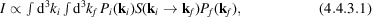where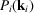is the probability that a neutron of wavevectoris incident on the sample,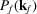is the probability that a neutron of wavevector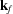is transmitted by the analyser system and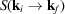is the probability that the sample scatters a neutron fromto. The fluctuation spectrum of the sample,, does not depend separately onandbut rather on the scattering vector Q and energy transferdefined by the conservation equations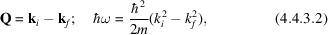where m is the neutron mass.

A number of methods of calculating the distribution functionsandhave been proposed. The method of independent distributions was used implicitly by Stedman (1968) and in more detail by Bjerrum Møller & Nielson (MN) (Nielsen & Bjerrum Møller, 1969; Bjerrum Møller & Nielsen, 1970) for three-axis spectrometers. Subsequently, the method has been extended to perfect-crystal monochromators (Pynn, Fujii & Shirane, 1983) and to time-of-flight spectrometers (Steinsvoll, 1973; Robinson, Pynn & Eckert, 1985). The method involves separating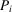and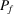into a product of independent distribution functions each of which can be convolved separately with the fluctuation spectrum S(Q, ω) [cf. equation (4.4.3.1)]. Extremely simple results are obtained for the widths of scans through a phonon dispersion surface for spectrometers where the energy of scattered neutrons is analysed (Nielson & Bjerrum Møller, 1969). For diffractometers, the width of a scan through a Bragg peak may also be obtained (Pynn et al., 1983), yielding a result equivalent to that given by Caglioti, Paoletti & Ricci (1960). In this case, however, the singular nature of the Bragg scattering process introduces a correlation between the distribution functions that contribute toandand the calculation is less transparent than it is for phonons.

A somewhat different approach, which does not explicitly separate the various contributions to the resolution, was proposed by Cooper & Nathans (CN) (Cooper & Nathans, 1967, 1968; Cooper, 1968). Minor errors were corrected by several authors (Werner & Pynn, 1971; Chesser & Axe, 1973). The CN method calculates the instrumental resolution function R(QQ0, ω − ω0) as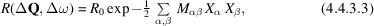where,, andare the three components of ΔQ, X4 = Δω, and Q0 and ω0 are obtained from (4.4.3.2)by replacingandbyand, respectively. The matrix M is given in explicit form by several authors (Cooper & Nathans, 1967, 1968; Cooper, 1968; Werner & Pynn, 1971; Chesser & Axe, 1973) and the normalization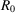has been discussed in detail by Dorner (1972). [A refutation (Tindle, 1984) of Dorner's work is incorrect.] Equation (4.4.3.3)implies that contours of constant transmission for the spectrometer [RQ, Δω) = constant] are ellipsoids in the four-dimensional Q–ω space. Optimum resolution (focusing) is achieved by a scan that causes the resolution function to intersect the feature of interest in S(Q, ω) (e.g. Bragg peak or phonon dispersion surface) for the minimum scan interval. The optimization of scans for a diffractometer has been considered by Werner (1971).

The MN and CN methods are equivalent. Using the MN formalism, it can be shown that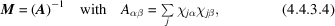where the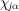are the components of the standard deviations of independent distributions (labelled by index j) defined by Bjerrum Møller & Nielsen (1970). In the limit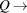0, the matrices M and A are of rank three and other methods must be used to calculate the resolution ellipsoid (Mitchell, Cowley & Higgins, 1984). Nevertheless, the MN method may be used even in this case to calculate widths of scans.

To obtain the resolution function of a diffractometer (in which there is no analysis of scattered neutron energy) from the CN form for M, it is sufficient to set to zero those contributions that arise from the mosaic of the analyser crystal. For elastic Bragg scattering, the problem is further simplified because[cf. equation (4.4.3.3)] is zero. The spectrometer resolution function is then an ellipsoid in Q space.

For the measurement of integrated intensities (of Bragg peaks for example), the normalizationin (4.4.3.3)is required in order to obtain the Lorentz factor. The latter has been calculated for an arbitrary scan of a three-axis spectrometer (Pynn, 1975) and the results may be modified for a diffractometer as described in the preceding paragraph.

### References

Bjerrum Møller, H. & Nielson, M. (1970). Proceedings of IAEA panel meeting on instrumentation for neutron inelastic scattering research. Vienna: IAEA.Google Scholar
Caglioti, G., Paoletti, A. & Ricci, F. P. (1960). On resolution and luminosity of a neutron diffraction spectrometer for single crystal analysis. Nucl. Instrum. Methods, 9, 195–198.Google Scholar
Chesser, N. J. & Axe, J. D. (1973). Derivation and experimental verification of the normalised resolution function for inelastic neutron scattering. Acta Cryst. A29, 160–169.Google Scholar
Cooper, M. J. (1968). The resolution function in neutron diffractometry. IV. Application of the resolution function to the measurement of Bragg peaks. Acta Cryst. A24, 624–627.Google Scholar
Cooper, M. J. & Nathans, R. (1967). The resolution function in neutron diffractometry. I. The resolution function of a neutron diffractometer and its application to phonon measurements. Acta Cryst. 23, 357–367.Google Scholar
Cooper, M. J. & Nathans, R. (1968). The resolution function in neutron diffractometry. II. Experimental determination and properties of the `elastic two-crystal' resolution function. Acta Cryst. A24, 619–624.Google Scholar
Dorner, B. (1972). The normalization of the resolution function for inelastic neutron scattering and its applications. Acta Cryst. A28, 319–327.Google Scholar
Mitchell, P. W., Cowley, R. A. & Higgins, S. A. (1984). The resolution function of triple-axis neutron spectrometers in the limit of small scattering angles. Acta Cryst. A40, 152–160.Google Scholar
Nielsen, M. & Bjerrum Møller, H. (1969). Resolution of a triple-axis spectrometer. Acta Cryst. A25, 547–550.Google Scholar
Pynn, R. (1975). Lorentz factor for triple-axis spectrometers. Acta Cryst. B31, 2555–2556.Google Scholar
Pynn, R. (1984). Reactor based neutron scattering instrumentation. Rev. Sci. Instrum. 55, 837–848.Google Scholar
Pynn, R., Fujii, Y. & Shirane, G. (1983). The resolution function of a perfect-crystal three-axis X-ray spectrometer. Acta Cryst. A39, 38–46. [There is an omission in equation (A3) of this reference; the relation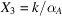should be added.]Google Scholar
Robinson, R. A., Pynn, R. & Eckert, J. (1985). An improved constant-Q spectrometer for pulsed neutron sources. Nucl. Instrum. Methods, A241, 312–324.Google Scholar
Stedman, R. (1968). Energy resolution and focusing in inelastic scattering experiments. Rev. Sci. Instrum. 39, 878–883.Google Scholar
Steinsvoll, O. (1973). The resolution function of a hybrid neutron spectrometer. Nucl. Instrum. Methods, 106, 453–459.Google Scholar
Tindle, G. L. (1984). A new instrumental factor in triple-axis spectrometry and Bragg reflectivity measurements. Acta Cryst. A40, 103–107.Google Scholar
Werner, S. A. (1971). Choice of scans in neutron diffraction. Acta Cryst. A27, 665–669.Google Scholar
Werner, S. A. & Pynn, R. (1971). Resolution effects in the measurement of phonons in sodium metal. J. Appl. Phys. 42, 4736–4749.Google Scholar